Cumulative Distribution Calculators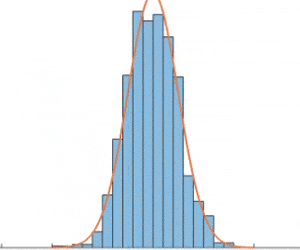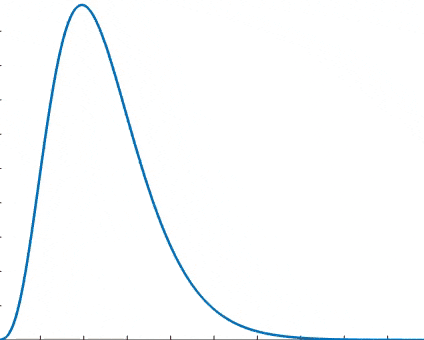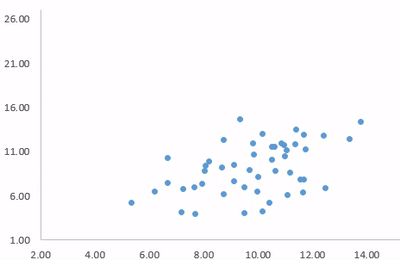Original: English

Here we have a set of cumulative probability calculators aiming to compute a probability for the following distribution functions: Normal, Lognormal, Gamma, Exponential, Student’s, Binomial, Geometric, Negative Binomial and Poisson. Information about these distributions are provided in the section "Summary of Distribution Functions" below in this page.

To calculate a probability, just follow the 3 steps below:

Step 1)  Select a probability distribution function:

Step 2)  Enter the parameter(s) for the selected distribution.

 Parameter 1 Parameter 2 Parameter 3

Step 3) Specify what you want to calculate:

I wish to know the odds of getting a number     than the value

Summary of Distribution Functions

The Cumulative Distribution Function (CDF) is the probability that the random variable X will take a value less than or equal to x. The following table summarizes common continuous and discrete distributions, showing the cumulative function and its parameters. In this table, the continuous distributions are: Normal, Lognormal, Gamma, Exponential, and Student’s. The discrete distributions are: Binomial, Geometric, Negative Binomial and Poisson.

 Normal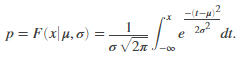Returns the probability 'p' that a single observation from a Normal distribution with parameters µ and σ falls in the interval (-∞,x]. The normal distribution is a two-parameter family of curves. The first parameter, µ, is the mean. The second parameter, σ, is the standard deviation. Lognormal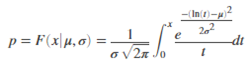Returns values at x of the Lognormal cumulative distribution function with distribution parameters µ and σ, where µ and σ are the mean and standard deviation, respectively, of the associated normal distribution.A lognormal distribution with mean m and variance v has parameters µ and σ. Gamma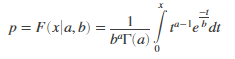Returns the Gamma cumulative distribution function at value x using the corresponding shape parameters in a and scale parameters in b. ExponentialComputes the Exponential cumulative distribution function at value x using the corresponding mean parameter µ. Student's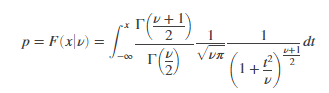Computes a Student’s cumulative distribution function at value x where ν is the degrees of freedom and Γ( · ) is the Gamma function. The result p is the probability that a single observation from the t distribution with ν degrees of freedom will fall in the interval [–∞, x]. Binomial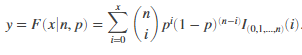Computes a binomial cumulative distribution function at value x using the corresponding number of trials in N and probability of success for each trial in p. Geometric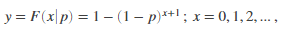Computes a Geometric cumulative distribution where p is the probability of success, and x is the number of failures before the first success. The result y is the probability of observing up to x trials before a success, when the probability of success in any given trial is p. Negative BinomialComputes the Negative binomial cumulative distribution at value x using the corresponding number of successes (R) and probability of success in a single trial (p). PoissonReturns the Poisson cumulative distribution function at value x using the corresponding mean parameter in lambda.

Remember you can access this website in your mobile phone and add it to your favorites.